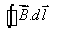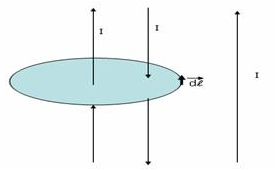Courses

# Test: Ampere’S Law

## 5 Questions MCQ Test Physics For JEE | Test: Ampere’S Law

Description
This mock test of Test: Ampere’S Law for JEE helps you for every JEE entrance exam. This contains 5 Multiple Choice Questions for JEE Test: Ampere’S Law (mcq) to study with solutions a complete question bank. The solved questions answers in this Test: Ampere’S Law quiz give you a good mix of easy questions and tough questions. JEE students definitely take this Test: Ampere’S Law exercise for a better result in the exam. You can find other Test: Ampere’S Law extra questions, long questions & short questions for JEE on EduRev as well by searching above.
QUESTION: 1

### A steady electric current is flowing through a cylindrical conductor.Then select the correct statement. 1. The electric field in the vicinity of the conductor is zero. 2. The magnetic field in the vicinity of the conductor is zero. 3. The electric field at the axis of the conductor is zero. 4. The magnetic field at the axis of the conductor is zero.​

Solution:

If a current passes through any conductor, net charge through any section is zero. hence, electric field in the vicinity of the conductor is zero. We can observe using Biot and Savart law that the magnetic field at the axis of the cylindrical wire will be zero. Electric field at the axis of the wire cannot be zero otherwise the current through the wire cannot flow.
So statement 1 and 4 are correct.

QUESTION: 2

### A current I ampere flows along an infinitely long straight thin-walled tube, then the magnetic induction at any point inside the tube is

Solution:

Changes(if required):
Solution:
Sinθ=0 on any pt. on the axis of conductor carrying current
B=(μo/4Π)​​i(dlSinθ​/r2)

QUESTION: 3

### If a long hollow copper pipe carries a direct current, the magnetic field associated with the current will be

Solution:

For a loop inside the hollow portion of the conductor, the current enclosed is zero until the loop is on the surface or inside the material of the conductor.
∴Binside=0
For any loop outside, current enclosed by the amperian loop is not zero, hence the magnetic field is non-zero.

QUESTION: 4

Ampere’s circuital law is analogous to a law in electrostatics.That law is

Solution:

Ampere's law is similar to Gauss's law in electrostatics.
Gauss's law correlates the surface integral of electric field E with charge q over a surface.
Ampere's law co-relates the line integral of magnetic field B with current over a closed path.

QUESTION: 5

In the figure shown, what is? The magnitude of current is the same in all three wires.Solution:

According to ampere law for closed loop.as the net current in the loop is zero therefore answer is zero
Let upward direction of the current be positive in sign
therefore that for downward direction will negative
B.dl=u × current inside the loop
=u×(+I-I)
=0